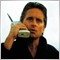# MAs cross well but do not make right trades18

```//Variable externas
extern double Lote = 0.01;
extern double StopLoss = 300;
extern double TakeProfit = 400;
extern int TrailingStop = 280;

extern int Slipagge = 10;
extern int MagicNumber = 12345;

extern int PeriodoEMARapidaActual = 5;
extern int PeriodoEMALentaActual = 21;

//Variables Globales
int TicketCompra;
int MaximoDeOrdenes = 2;
int BarsCount = 0;
int i;
bool fm;

//+------------------------------------------------------------------+
//| Expert initialization function                                   |
//+------------------------------------------------------------------+
void OnTick()
{
//Medias Exponenciales
double EMARapidaActual = iMA(NULL,0,PeriodoEMARapidaActual,0,1,0,1);
double EMALentaActual = iMA(NULL,0,PeriodoEMALentaActual,0,1,0,1);

//Orden de compra                                          // Linea EMAs: "EMARapida es mayor a EMALenta y no hay error

if (OrdersTotal()==MaximoDeOrdenes)

if (Bars > BarsCount)
//(x && y) = Si x e y son verdaderos, la expresion es verdadera
{                                                          //(x == y) = x es igual a y    0 = No error returned
//Abrir operacion de compra

BarsCount = Bars;
}

```

Hi guys,

What I want to do is that the EA open Buy trades when the 5 EMA crosses over the 21 EMA, BUT also when the distance bewtween the two EMA's is not decreasing.

Other condition that could make me sense, is that the two EMA's must be increasing (Regardless the distance between them).

Any suggestion is welcome,

Thanks!
Files:38646

Moved to MT4 section.20207

SalgadoCapital: What I want to do is that the EA open Buy trades when the 5 EMA crosses over the 21 EMA, BUT also when the distance bewtween the two EMA's is not decreasing.

Contradictory statements. Increasing distance is not a cross. Your code doesn't test for either.